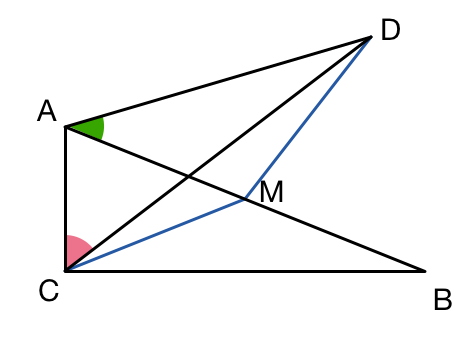# A geometry problem by Chung Kevin

Geometry Level 1$ABC$ is a right triangle with $\angle ACB=90^\circ$. $M$ is the midpoint of $AB,$ and $D$ is a point such that $MC = MD$ with points $C$ and $D$ lying on opposite sides of $AB,$ as shown in the diagram.

If $\angle DAB = 40 ^ \circ$, what is $\angle DCA$?

×